1572330709

# Introduction to Image Segmentation with K-Means clustering

Image segmentation is an important step in image processing, and it seems everywhere if we want to analyze what’s inside the image. For example, if we seek to find if there is a chair or person inside an indoor image, we may need image segmentation to separate objects and analyze each object individually to check what it is. Image segmentation usually serves as the pre-processing before pattern recognition, feature extraction, and compression of the image.

Image segmentation is the classification of an image into different groups. Many kinds of research have been done in the area of image segmentation using clustering. There are different methods and one of the most popular methods is K-Means clustering algorithm.

So here in this article, we will explore a method to read an image and cluster different regions of the image. But before doing lets first talk about:

1. Image Segmentation
2. How Image segmentation works
3. K-Means clustering ML Algorithm
4. Merge K-Means clustering Algorithm with Image Segmentation.
5. Canny Edge detection

## Image Segmentation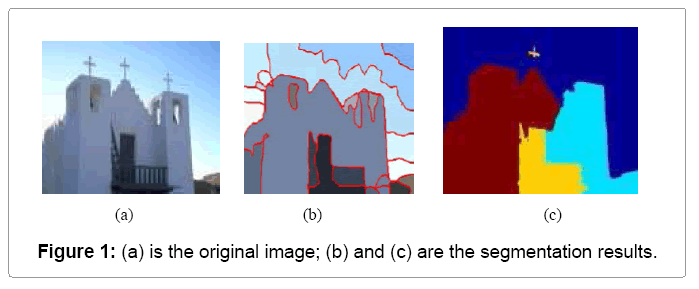Image segmentation is the process of partitioning a digital image into multiple distinct regions containing each pixel(sets of pixels, also known as superpixels) with similar attributes.

The goal of Image segmentation is to change the representation of an image into something that is more meaningful and easier to analyze.

Image segmentation is typically used to locate objects and boundaries (lines, curves, etc.) in images. More precisely, Image Segmentation is the process of assigning a label to every pixel in an image such that pixels with the same label share certain characteristics.

Of course, a common question arises:

Why does Image Segmentation even matter?

If we take an example of Autonomous Vehicles, they need sensory input devices like cameras, radar, and lasers to allow the car to perceive the world around it, creating a digital map. Autonomous driving is not even possible without object detection which itself involves image classification/segmentation.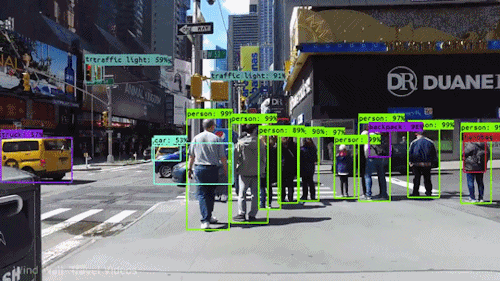Object detection and Image Classification by an Autonomous Vehicle

Other examples involve Healthcare Industry where if we talk about Cancer, even in today’s age of technological advancements, cancer can be fatal if we don’t identify it at an early stage. Detecting cancerous cell(s) as quickly as possible can potentially save millions of lives. The shape of the cancerous cells plays a vital role in determining the severity of cancer which can be identified using image classification algorithms.

Like this, there were several algorithms and techniques for image segmentation have been developed over the years using domain-specific knowledge to effectively solve segmentation problems in that specific application area which includes medical imaging, object detection, Iris recognition, video surveillance, machine vision and many more….

Let us plot an image in 3D space using python matplotlib library.

Below is the image that we’ll gonna plot in 3D space and we can clearly see 3 different colors which means 3 clusters/groups should be generated.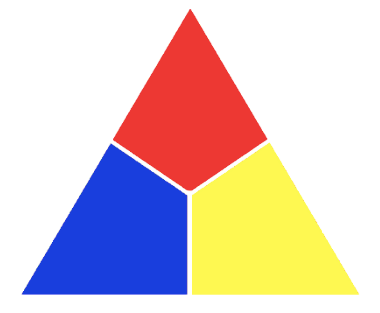``````import matplotlib.pyplot as plt
from mpl_toolkits.mplot3d import Axes3D
img = cv2.cvtColor(img, cv2.COLOR_BGR2RGB)
r, g, b = cv2.split(img)
r = r.flatten()
g = g.flatten()
b = b.flatten()#plotting
fig = plt.figure()
ax = Axes3D(fig)
ax.scatter(r, g, b)
plt.show()
``````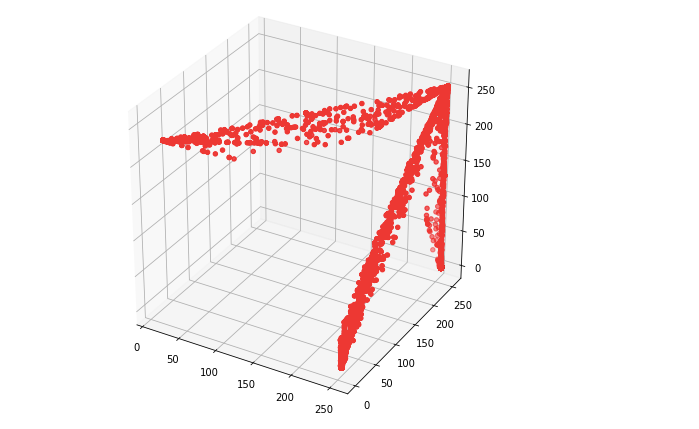From the plot one can easily see that the data points are forming groups — some places in a graph are more dense, which we can think as different colors’ dominance on the image.

## How Image Segmentation works

Image Segmentation involves converting an image into a collection of regions of pixels that are represented by a mask or a labeled image. By dividing an image into segments, you can process only the important segments of the image instead of processing the entire image.

A common technique is to look for abrupt discontinuities in pixel values, which typically indicate edges that define a region.

Another common approach is to detect similarities in the regions of an image. Some techniques that follow this approach are region growing, clustering, and thresholding.

A variety of other approaches to perform image segmentation have been developed over the years using domain-specific knowledge to effectively solve segmentation problems in specific application areas.

So let us start with one of the clustering-based approaches in Image Segmentation which is K-Means clustering.

## K-Means clustering algorithm

Ok first What are Clustering algorithms in Machine Learning?

Clustering algorithms are unsupervised algorithms but are similar to Classification algorithms but the basis is different.

In Clustering, you don’t know what you are looking for, and you are trying to identify some segments or clusters in your data. When you use clustering algorithms in your dataset, unexpected things can suddenly pop-up like structures, clusters, and groupings you would have never thought otherwise.

K-Means clustering algorithm is an unsupervised algorithm and it is used to segment the interest area from the background. It clusters, or partitions the given data into K-clusters or parts based on the K-centroids.

The algorithm is used when you have unlabeled data(i.e. data without defined categories or groups). The goal is to find certain groups based on some kind of similarity in the data with the number of groups represented by K.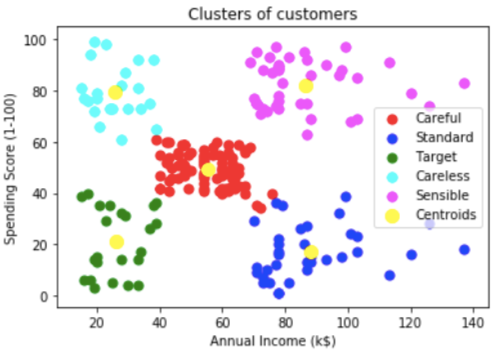In the above figure, Customers of a shopping mall have been grouped into 5 clusters based on their income and spending score. Yellow dots represent the Centroid of each cluster.

The objective of K-Means clustering is to minimize the sum of squared distances between all points and the cluster center.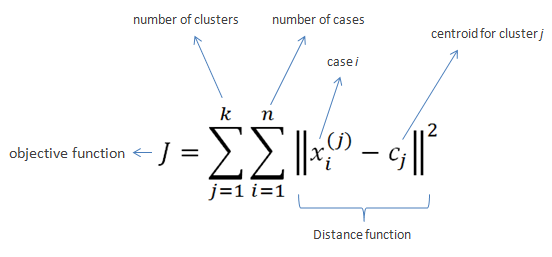Steps in K-Means algorithm: 1. Choose the number of clusters K.
2. Select at random K points, the centroids(not necessarily from your dataset).
3. Assign each data point to the closest centroid → that forms K clusters.
4. Compute and place the new centroid of each cluster.
5. Reassign each data point to the new closest centroid. If any reassignment . took place, go to step 4, otherwise, the model is ready.

How to choose the optimal value of K?

For a certain class of clustering algorithms (in particular K-Means, K-medoids, and expectation-maximization algorithm), there is a parameter commonly referred to as K that specifies the number of clusters to detect. Other algorithms such as DBSCAN and OPTICS algorithm do not require the specification of this parameter; Hierarchical Clustering avoids the problem altogether but that’s beyond the scope of this article.

If we talk about K-Means then the correct choice of K is often ambiguous, with interpretations depending on the shape and scale of the distribution of points in a data set and the desired clustering resolution of the user. In addition, increasing K without penalty will always reduce the amount of error in the resulting clustering, to the extreme case of zero error if each data point is considered its own cluster (i.e., when K equals the number of data points, n). Intuitively then, the optimal choice of K will strike a balance between maximum compression of the data using a single cluster, and maximum accuracy by assigning each data point to its own cluster.

If an appropriate value of K is not apparent from prior knowledge of the properties of the data set, it must be chosen somehow. There are several categories of methods for making this decision and Elbow method is one such method.

## Elbow method

The basic idea behind partitioning methods, such as K-Means clustering, is to define clusters such that the total intra-cluster variation or in other words, total within-cluster sum of square (WCSS) is minimized. The total WCSS measures the compactness of the clustering and we want it to be as small as possible.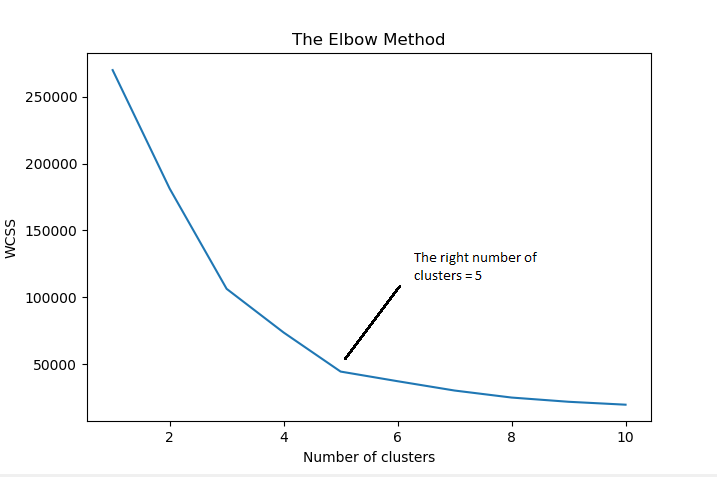The Elbow method looks at the total WCSS as a function of the number of clusters: One should choose a number of clusters so that adding another cluster doesn’t improve much better the total WCSS.

Steps to choose the optimal number of clusters K:(Elbow Method) 1. Compute K-Means clustering for different values of K by varying K from 1 to 10 clusters.
2. For each K, calculate the total within-cluster sum of square (WCSS).
3. Plot the curve of WCSS vs the number of clusters K.
4. The location of a bend (knee) in the plot is generally considered as an indicator of the appropriate number of clusters.

There is a catch!!!

In spite of all the advantages K-Means have got but it fails sometimes due to the random choice of centroids which is called The Random Initialization Trap.

To solve this issue we have an initialization procedure for K-Means which is called K-Means++(Algorithm for choosing the initial values for K-Means clustering).

In K-Means++, We pick a point randomly and that’s your first centroid, then we pick the next point based on the probability that depends upon the distance of the first point, the further apart the point is the more probable it is.

Then we have two centroids, repeat the process, the probability of each point is based on its distance to the closest centroid to that point. Now, this introduces an overhead in the initialization of the algorithm, but it reduces the probability of a bad initialization leading to bad clustering result.

Visual Representation of K-Means Clustering: Starting with 4 leftmost points.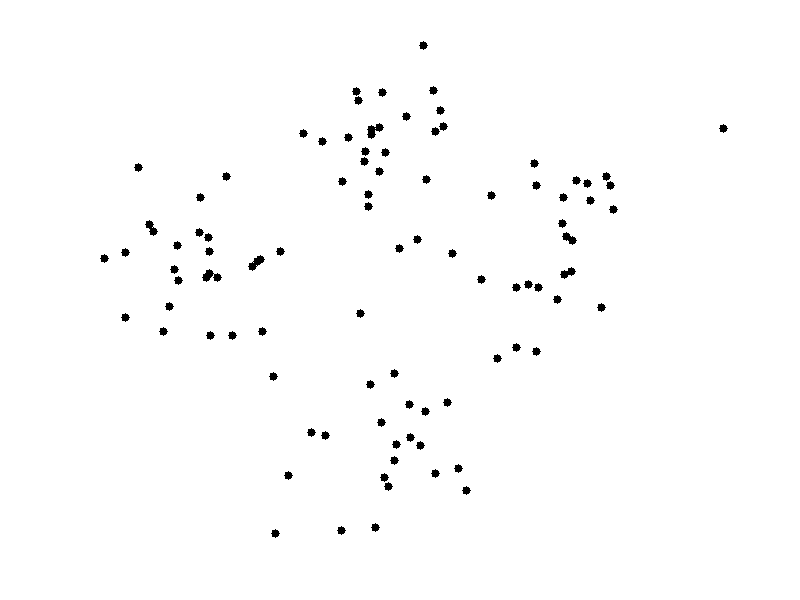Enough of theory lets implement what we have discussed in a real-world scenario.

In this section, we will explore a method to read an image and cluster different regions of the image using the K-Means clustering algorithm and OpenCV.

So basically we will perform Color clustering and Canny Edge detection.

Color Clustering:

``````import numpy as np
import cv2
import matplotlib.pyplot as plt
``````

Next step is to load the image in RGB color space

``````original_image = cv2.imread("/Users/nageshsinghchauhan/Desktop/image1.jpg")
``````

Original Image: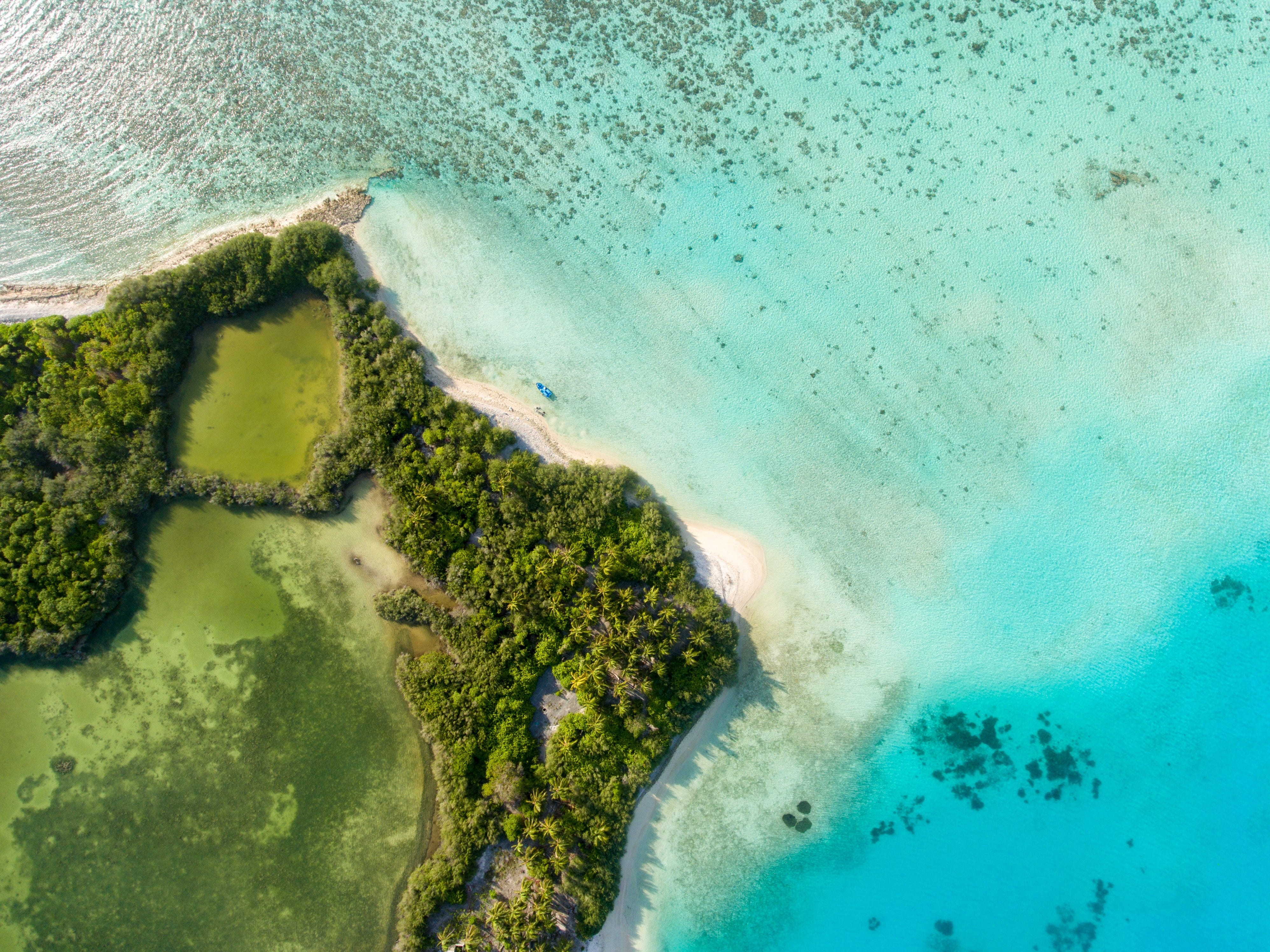We need to convert our image from RGB Colours Space to HSV to work ahead.

But the question is why ??

According to wikipedia the R, G, and B components of an object’s color in a digital image are all correlated with the amount of light hitting the object, and therefore with each other, image descriptions in terms of those components make object discrimination difficult. Descriptions in terms of hue/lightness/chroma or hue/lightness/saturation are often more relevant.

If you don’t convert your image to HSV, your image will look something like this: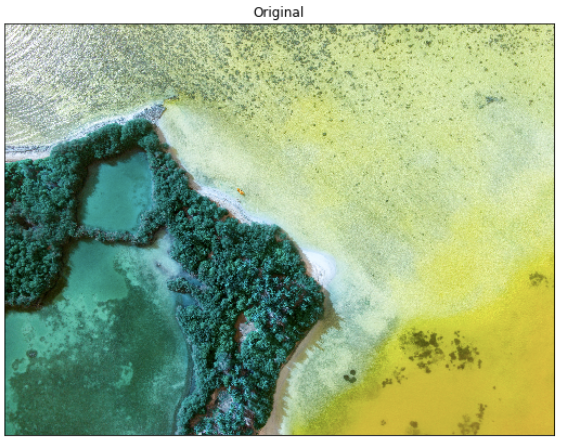``````img=cv2.cvtColor(original_image,cv2.COLOR_BGR2RGB)
``````

Next, converts the MxNx3 image into a Kx3 matrix where K=MxN and each row is now a vector in the 3-D space of RGB.

``````vectorized = img.reshape((-1,3))
``````

We convert the unit8 values to float as it is a requirement of the k-means method of OpenCV.

``````vectorized = np.float32(vectorized)
``````

We are going to cluster with k = 3 because if you look at the image above it has 3 colors, green-colored grass and forest, blue sea and the greenish-blue seashore.

Define criteria, number of clusters(K) and apply k-means()

``````criteria = (cv2.TERM_CRITERIA_EPS + cv2.TERM_CRITERIA_MAX_ITER, 10, 1.0)
``````

OpenCV provides cv2.kmeans(samples, nclusters(K), criteria, attempts, flags)function for color clustering.

1. samples: It should be of np.float32 data type, and each feature should be put in a single column.

2. nclusters(K): Number of clusters required at the end

3. criteria: It is the iteration termination criteria. When this criterion is satisfied, the algorithm iteration stops. Actually, it should be a tuple of 3 parameters. They are `( type, max_iter, epsilon )`:

Type of termination criteria. It has 3 flags as below:

• cv.TERM_CRITERIA_EPS — stop the algorithm iteration if specified accuracy, epsilon, is reached.
• cv.TERM_CRITERIA_MAX_ITER — stop the algorithm after the specified number of iterations, max_iter.
• cv.TERM_CRITERIA_EPS + cv.TERM_CRITERIA_MAX_ITER — stop the iteration when any of the above condition is met.

4. attempts: Flag to specify the number of times the algorithm is executed using different initial labelings. The algorithm returns the labels that yield the best compactness. This compactness is returned as output.

5. flags: This flag is used to specify how initial centers are taken. Normally two flags are used for this: cv.KMEANS_PP_CENTERS and cv.KMEANS_RANDOM_CENTERS.

``````K = 3
attempts=10
ret,label,center=cv2.kmeans(vectorized,K,None,criteria,attempts,cv2.KMEANS_PP_CENTERS)
``````

Now convert back into uint8.

``````center = np.uint8(center)
``````

Next, we have to access the labels to regenerate the clustered image

``````res = center[label.flatten()]
result_image = res.reshape((img.shape))
``````

`result_image` is the result of the frame which has undergone k-means clustering.

Now let us visualize the output result with K=3

``````figure_size = 15
plt.figure(figsize=(figure_size,figure_size))
plt.subplot(1,2,1),plt.imshow(img)
plt.title('Original Image'), plt.xticks([]), plt.yticks([])
plt.subplot(1,2,2),plt.imshow(res2)
plt.title('Segmented Image when K = %i' % K), plt.xticks([]), plt.yticks([])
plt.show()
``````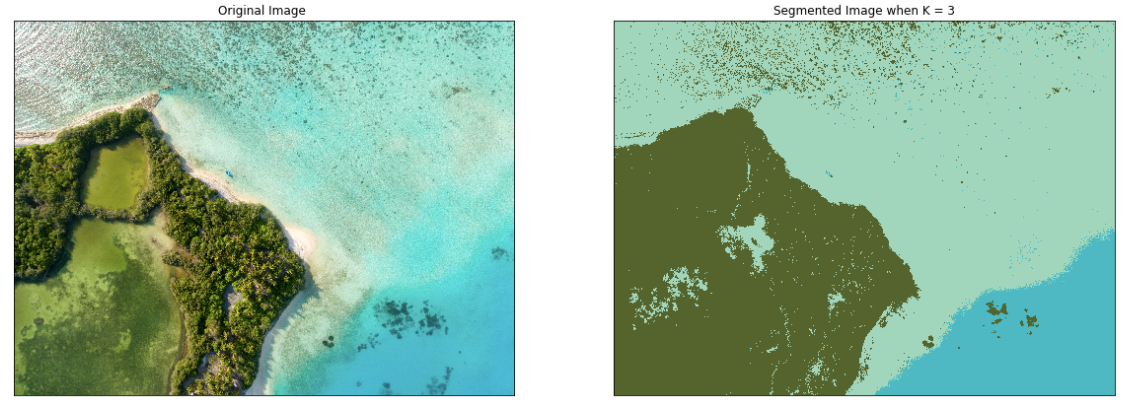So the algorithm has categorized our original image into three dominant colors.

Let’s see what happens when we change the value of K=5: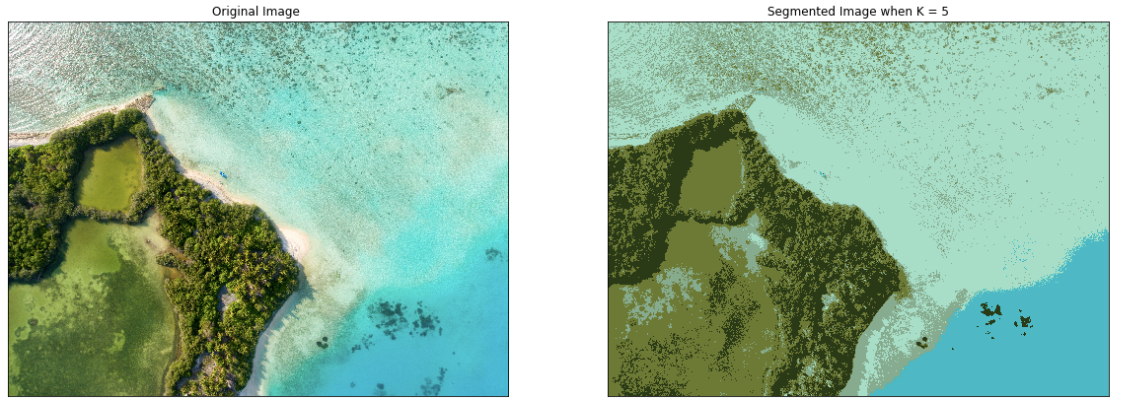Change the value of K=7: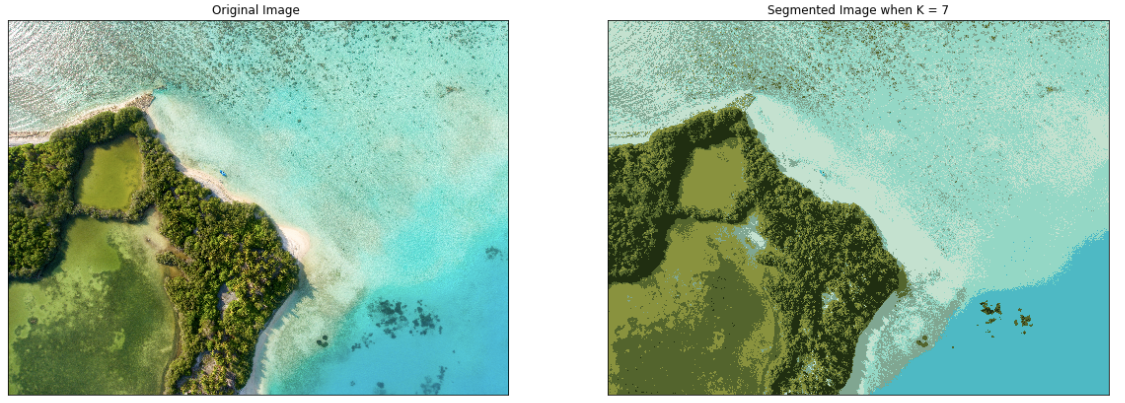As you can see with an increase in the value of K, the image becomes clearer because the K-means algorithm can classify more classes/cluster of colors.

We can try our code for different images: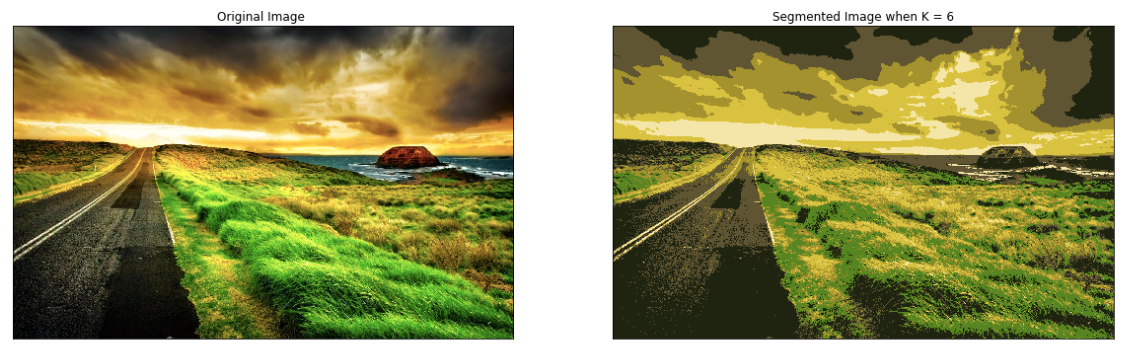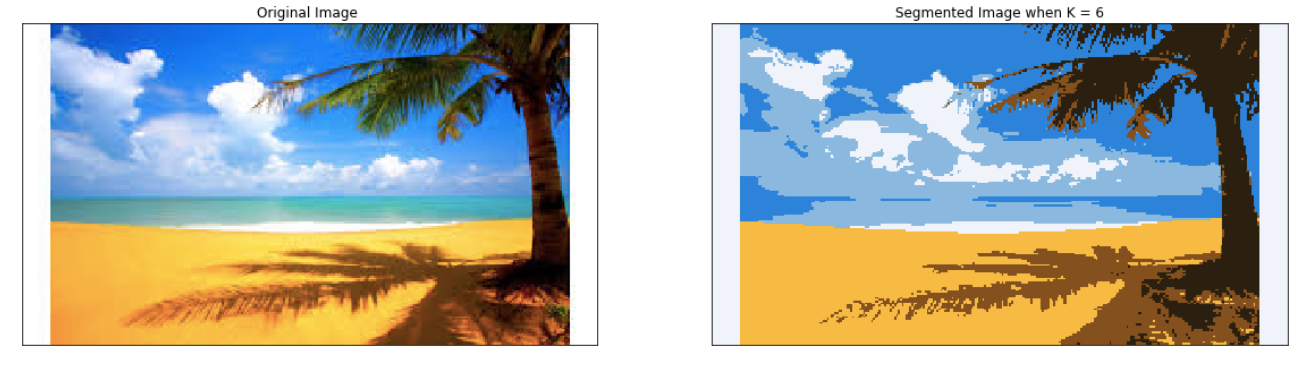Let’s move to our next part which is Canny Edge detection.

Canny Edge detection: It is an image processing method used to detect edges in an image while suppressing noise.

The Canny Edge detection algorithm is composed of 5 steps: 1.Noise reduction
3. Non-maximum suppression
4. Double threshold
5. Edge Tracking by Hysteresis

OpenCV provides cv2.Canny(image, threshold1,threshold2) function for edge detection.

The first argument is our input image. Second and third arguments are our min and max threshold respectively.

The function finds edges in the input image(8-bit input image) and marks them in the output map edges using the Canny algorithm. The smallest value between threshold1 and threshold2 is used for edge linking. The largest value is used to find initial segments of strong edges.

``````edges = cv2.Canny(img,150,200)
plt.figure(figsize=(figure_size,figure_size))
plt.subplot(1,2,1),plt.imshow(img)
plt.title('Original Image'), plt.xticks([]), plt.yticks([])
plt.subplot(1,2,2),plt.imshow(edges,cmap = 'gray')
plt.title('Edge Image'), plt.xticks([]), plt.yticks([])
plt.show()
``````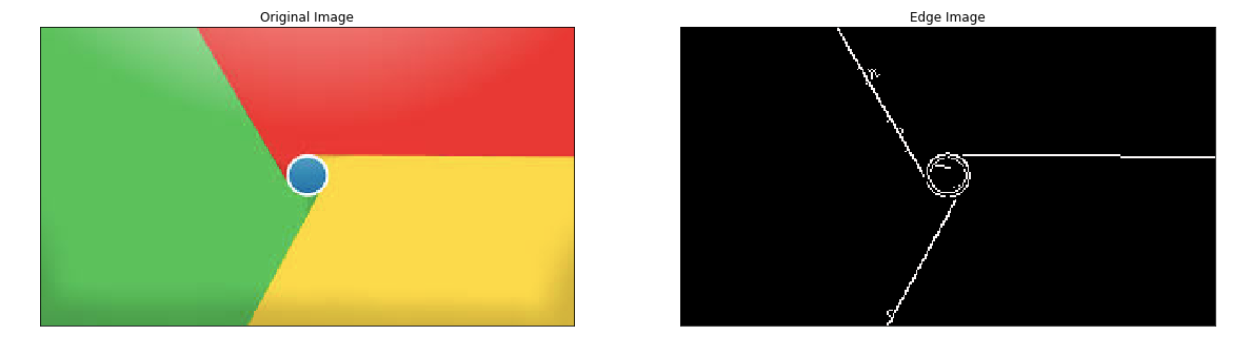Result-1: Edge detection using the Canny algorithm ![Introduction to Image Segmentation with K-Means clustering](https://miro.medium.com/max/1232/1*3tObJxCcj8NZkjplOjgeiA.png "Introduction to Image Segmentation with K-Means clustering")

Result-2: Edge detection using the Canny algorithm

## Conclusion: What the future holds

Due to advancements in Image processing, Machine learning, AI and related technologies, there will be millions of robots in the world in a few decades time, transforming the way we live our daily lives. These advancements will involve spoken commands, anticipating the information requirements of governments, translating languages, recognizing and tracking people and things, diagnosing medical conditions, performing surgery, reprogramming defects in human DNA, driverless cars and many more applications, the count of real-life applications is endless.

#machine-learning #data-science

## Buddha Community1597766400

## Context

I have been working in Advertising, specifically Digital Media and Performance, for nearly 3 years and customer behaviour analysis is one of the core concentrations in my day-to-day job. With the help of different analytics platforms (e.g. Google Analytics, Adobe Analytics), my life has been made easier than before since these platforms come with the built-in function of segmentation that analyses user behaviours across dimensions and metrics.

However, despite the convenience provided, I was hoping to leverage Machine Learning to do customer segmentation that can be scalable and applicable to other optimizations in Data Science (e.g. A/B Testing). Then, I came across the dataset provided by Google Analytics for a Kaggle competition and decided to use it for this project.

Feel free to check out the dataset here if you’re keen! Beware that the dataset has several sub-datasets and each has more than 900k rows!

## A. Explanatory Data Analysis (EDA)

This always remain an essential step in every Data Science project to ensure the dataset is clean and properly pre-processed to be used for modelling.

First of all, let’s import all the necessary libraries and read the csv file:

``````import pandas as pd
import matplotlib.pyplot as plt
import numpy as np
import seaborn as sns
``````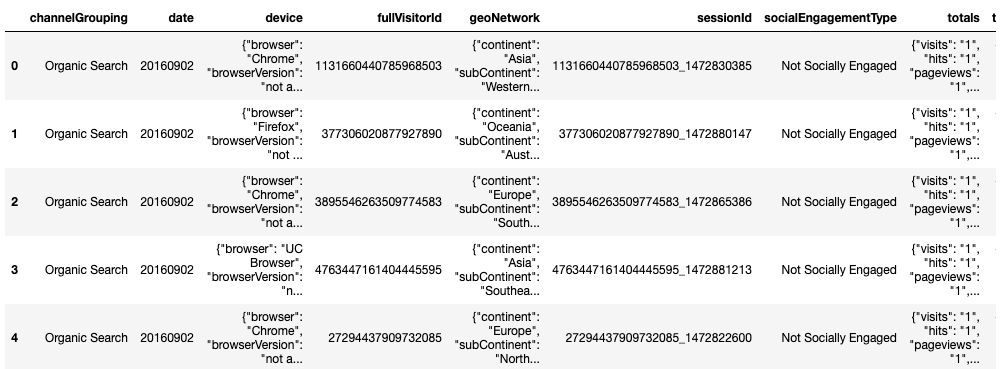### 1. Flatten JSON Fields

As you can see, the raw dataset above is a bit “messy” and not digestible at all since some variables are formatted as JSON fields which compress different values of different sub-variables into one field. For example, for geoNetwork variable, we can tell that there are several sub-variables such as continent, subContinent, etc. that are grouped together.

Thanks to the help of a Kaggler, I was able to convert these variables to a more digestible ones by flattening those JSON fields:

``````import os
import json
from pandas import json_normalize

json_columns = ['device', 'geoNetwork', 'totals', 'trafficSource']
for column in json_columns:
column_converted = json_normalize(df[column])
column_converted.columns = [f"{column}_{subcolumn}" for subcolumn in column_converted.columns]
df = df.drop(column, axis=1).merge(column_converted, right_index=True, left_index=True)
return df
``````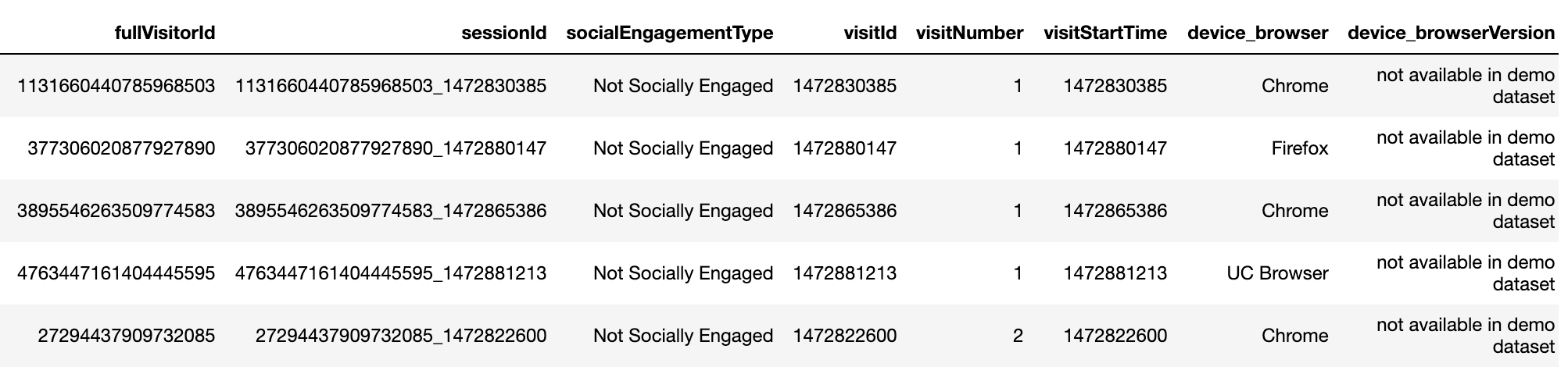After flattening those JSON fields, we are able to see a much cleaner dataset, especially those JSON variables split into sub-variables (e.g. device split into device_browser, device_browserVersion, etc.).

### 2. Data Re-formatting & Grouping

For this project, I have chosen the variables that I believe have better impact or correlation to the user behaviours:

``````df = df.loc[:,['channelGrouping', 'date', 'fullVisitorId', 'sessionId', 'visitId', 'visitNumber', 'device_browser', 'device_operatingSystem', 'device_isMobile', 'geoNetwork_country', 'trafficSource_source', 'totals_visits', 'totals_hits', 'totals_pageviews', 'totals_bounces', 'totals_transactionRevenue']]
df = df.fillna(value=0)
``````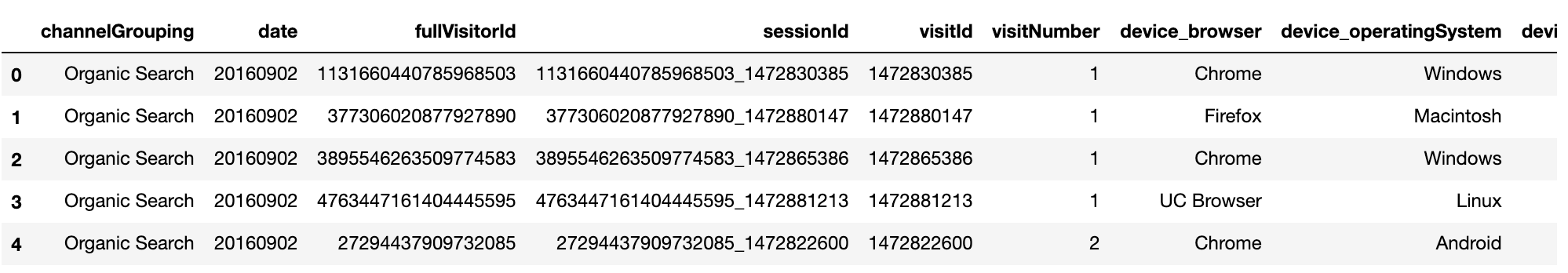Moving on, as the new dataset has fewer variables which, however, vary in terms of data type, I took some time to analyze each and every variable to ensure the data is “clean enough” prior to modelling. Below are some quick examples of un-clean data to be cleaned:

``````#Format the values
df.channelGrouping.unique()
df.channelGrouping = df.channelGrouping.replace("(Other)", "Others")

#Convert boolean type to string
df.device_isMobile.unique()
df.device_isMobile = df.device_isMobile.astype(str)
df.loc[df.device_isMobile == "False", "device"] = "Desktop"
df.loc[df.device_isMobile == "True", "device"] = "Mobile"
#Categorize similar values
df['traffic_source'] = df.trafficSource_source
df.traffic_source[df.traffic_source.str.contains("baidu")] = "baidu"
df.traffic_source[df.traffic_source.str.contains("bing")] = "bing"
df.traffic_source[df.traffic_source.str.contains("yahoo")] = "yahoo"
.....
df.traffic_source[~df.traffic_source.isin(main_traffic_source)] = "Others"
``````

After re-formatting, I found that fullVisitorID’s unique values are fewer than the total rows of the dataset, meaning there are multiple fullVisitorIDs that were recorded. Hence, I proceeded to group the variables by fullVisitorID and sort by Revenue:

``````df_groupby = df.groupby(['fullVisitorId', 'channelGrouping', 'geoNetwork_country', 'traffic_source', 'device', 'deviceBrowser', 'device_operatingSystem'])
.agg({'totals_hits':'sum', 'totals_pageviews':'sum', 'totals_bounces':'sum','totals_transactionRevenue':'sum'})
.reset_index()
df_groupby = df_groupby.sort_values(by='totals_transactionRevenue', ascending=False).reset_index(drop=True)
``````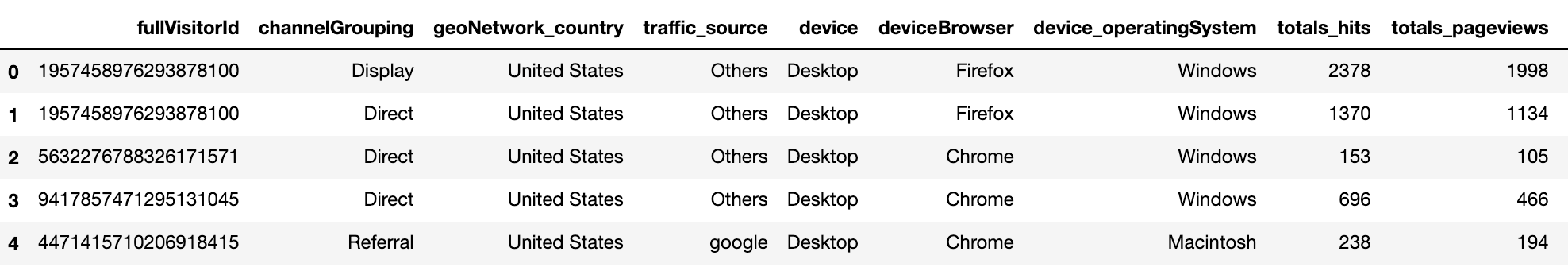#machine-learning #k-means #segmentation #data-science #clustering1600190040

## SciPy Cluster - K-Means Clustering and Hierarchical Clustering

SciPy is the most efficient open-source library in python. The main purpose is to compute mathematical and scientific problems. There are many sub-packages in SciPy which further increases its functionality. This is a very important package for data interpretation. We can segregate clusters from the data set. We can perform clustering using a single or multi-cluster. Initially, we generate the data set. Then we perform clustering on the data set. Let us learn more SciPy Clusters.

#### K-means Clustering

It is a method that can employ to determine clusters and their center. We can use this process on the raw data set. We can define a cluster when the points inside the cluster have the minimum distance when we compare it to points outside the cluster. The k-means method operates in two steps, given an initial set of k-centers,

• We define the cluster data points for the given cluster center. The points are such that they are closer to the cluster center than any other center.
• We then calculate the mean for all the data points. The mean value then becomes the new cluster center.

The process iterates until the center value becomes constant. We then fix and assign the center value. The implementation of this process is very accurate using the SciPy library.

#numpy tutorials #clustering in scipy #k-means clustering in scipy #scipy clusters #numpy1621443060

## Understanding Core Data Science Algorithms: K-Means and K-Medoids Clustering

This article provides an overview of core data science algorithms used in statistical data analysis, specifically k-means and k-medoids clustering.

Clustering is one of the major techniques used for statistical data analysis.

As the term suggests, “clustering” is defined as the process of gathering similar objects into different groups or distribution of datasets into subsets with a defined distance measure.

K-means clustering is touted as a foundational algorithm every data scientist ought to have in their toolbox. The popularity of the algorithm in the data science industry is due to its extraordinary features:

• Simplicity
• Speed
• Efficiency

#big data #big data analytics #k-means clustering #big data algorithms #k-means #data science algorithms1596381480

## Introduction to k-Means Clustering

#### What is Clustering?

Clustering is an unsupervised learning technique which is used to make clusters of objects i.e. it is a technique to group objects of similar kind in a group. In clustering, we first partition the set of data into groups based on the similarity and then assign the labels to those groups. Also, it helps us to find out various useful features that can help in distinguishing between different groups.

#### Types of Clustering

Most common categories of clustering are:-

• Partitioning Method
• Hierarchical Method
• Density-based Method
• Grid-based Method
• Model-based Method

#### Partitioning Method

Partitioning method classifies the group of n objects into groups based on the features and similarity of data.

The general problem would be like that we will have ‘n’ objects and we need to construct ‘k’ partitions among the data objects where each partition represents a cluster and will contain at least one object. Also, there is an additional condition that says each object can belong to only one group.

The partitioning method starts by creating an initial random partitioning. Then it iterates to improve the partitioning by moving the objects from one partition to another.

k-Means clustering follows the partitioning approach to classify the data.

#### Hierarchical Method

The hierarchical method performs a hierarchical decomposition of the given set of data objects. It starts by considering every data point as a separate cluster and then iteratively identifies two clusters which can be closest together and then merge these two clusters into one. We continue this until all the clusters are merged together into a single big cluster. A diagram called **Dendrogram **is used torepresent this hierarchy.

There are two approaches depending on how we create the hierarchy −

• Agglomerative Approach
• Divisive Approach

Agglomerative Approach

Agglomerative approach is a type of hierarchical method which uses bottom-up strategy. We start with each object considering as a separate cluster and keeps on merging the objects that are close to one another. It keep on doing so until all of the groups are merged into one or until the termination condition holds.

#k-means-clustering #machine-learning #clustering #python #code1595578740

## Using K-Means Clustering for Image Segregation

KMeans clustering is one of the most used unsupervised machine learning algorithms. As the name suggests, it can be used to create clusters of data, essentially segregating them.

Let’s get started. Here I will take a simple example to separate images from a folder that has both images of cats and dogs to their own clusters. This will create two separate folders (clusters). We will also go through how to automatically determine the optimal value for K.

I have generated a dataset of images of cats and dogs.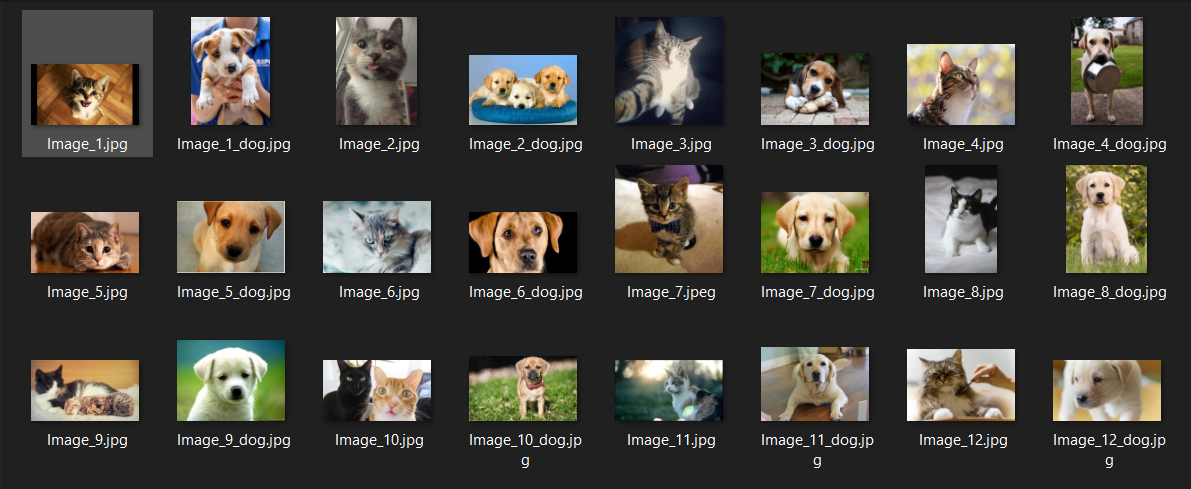Images of Cats and Dogs.

First off, we will start by importing the required libraries.

``````import numpy as np
import tensorflow as tf
import matplotlib.pyplot as plt
from sklearn.cluster import KMeans
from sklearn.metrics import silhouette_score
import cv2
import os, glob, shutil
``````

Then we will read all the images from the images folder and process them to extract for feature extraction. We will resize images to 224x224 to match the size of the input layer of our model for feature extraction.

``````input_dir = 'pets'
glob_dir = input_dir + '/*.jpg'

images = [cv2.resize(cv2.imread(file), (224, 224)) for file in glob.glob(glob_dir)]
paths = [file for file in glob.glob(glob_dir)]
images = np.array(np.float32(images).reshape(len(images), -1)/255)
``````

Now we will do feature extraction with the help of MobileNetV2 (Transfer Learning). Why MobileNetV2? You may ask. We can use ResNet50, InceptionV3, etc. but MobileNetV2 is fast and not so resource heavy so that’s my choice here.

``````model = tf.keras.applications.MobileNetV2(include_top=False,
weights=’imagenet’, input_shape=(224, 224, 3))

predictions = model.predict(images.reshape(-1, 224, 224, 3))
pred_images = predictions.reshape(images.shape, -1)
``````

Now that we have extracted the features, we can now do clustering by using KMeans. Since we already know that we are separating images of cats and dogs, with know the

``````k = 2
kmodel = KMeans(n_clusters = k, n_jobs=-1, random_state=728)
kmodel.fit(pred_images)
kpredictions = kmodel.predict(pred_images)
shutil.rmtree(‘output’)
for i in range(k):
os.makedirs(“output\cluster” + str(i))
for i in range(len(paths)):
shutil.copy2(paths[i], “output\cluster”+str(kpredictions[i]))
``````

#image-clustering #artificial-intelligence #machine-learning #transfer-learning #k-means #deep learning